Скачать презентацию Chapter 2 Introduction to Linear Programming Business Analytics

500cdfc95439292129753f2fd118472d.ppt

• Количество слайдов: 22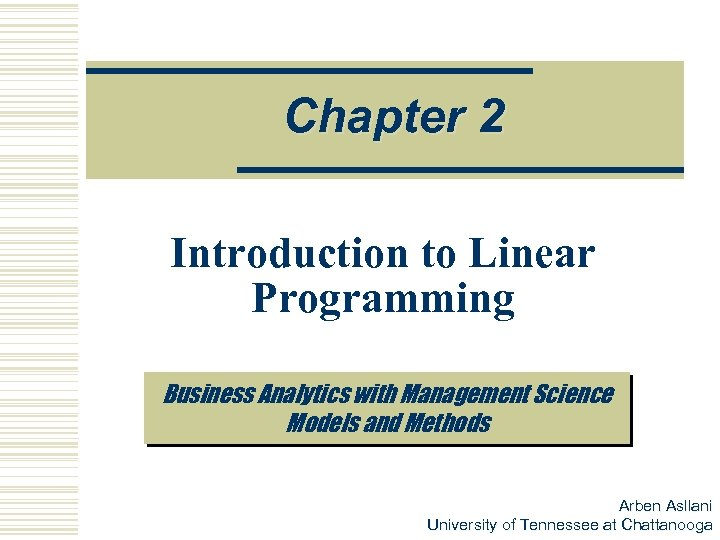Chapter 2 Introduction to Linear Programming Business Analytics with Management Science Models and Methods Arben Asllani University of Tennessee at Chattanooga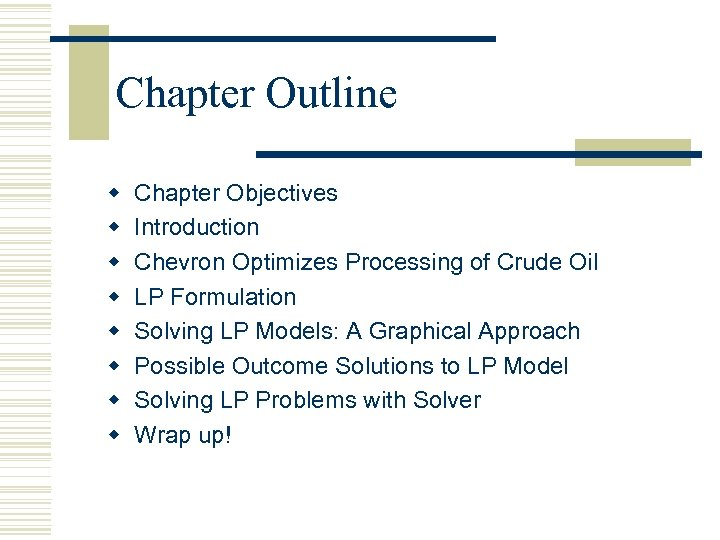Chapter Outline w w w w Chapter Objectives Introduction Chevron Optimizes Processing of Crude Oil LP Formulation Solving LP Models: A Graphical Approach Possible Outcome Solutions to LP Model Solving LP Problems with Solver Wrap up!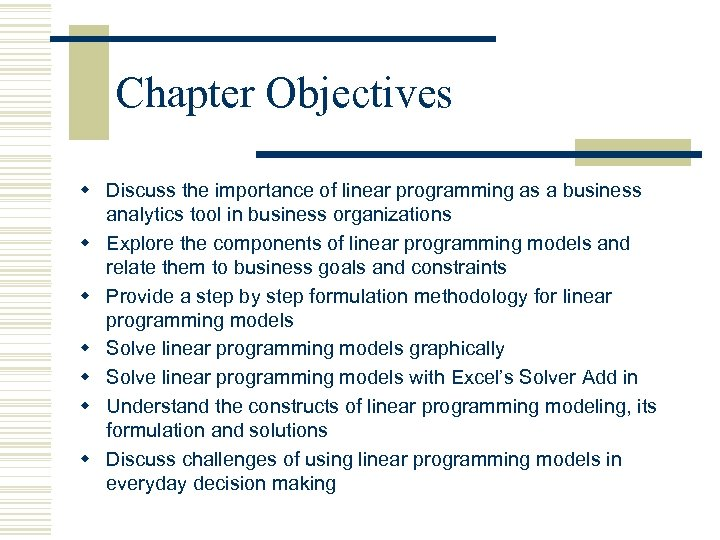Chapter Objectives w Discuss the importance of linear programming as a business analytics tool in business organizations w Explore the components of linear programming models and relate them to business goals and constraints w Provide a step by step formulation methodology for linear programming models w Solve linear programming models graphically w Solve linear programming models with Excel’s Solver Add in w Understand the constructs of linear programming modeling, its formulation and solutions w Discuss challenges of using linear programming models in everyday decision making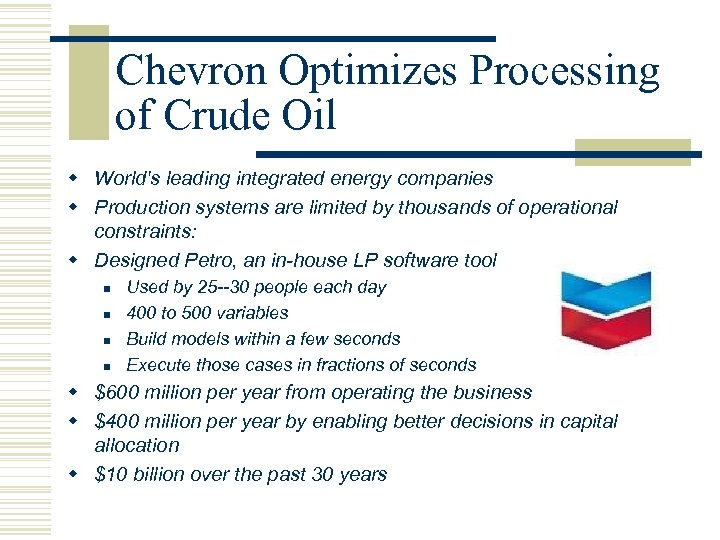Chevron Optimizes Processing of Crude Oil w World's leading integrated energy companies w Production systems are limited by thousands of operational constraints: w Designed Petro, an in-house LP software tool n n Used by 25 --30 people each day 400 to 500 variables Build models within a few seconds Execute those cases in fractions of seconds w \$600 million per year from operating the business w \$400 million per year by enabling better decisions in capital allocation w \$10 billion over the past 30 years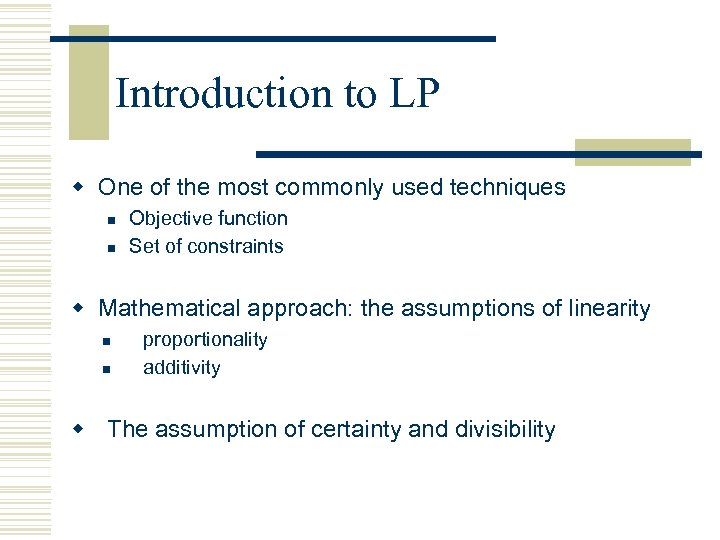Introduction to LP w One of the most commonly used techniques n n Objective function Set of constraints w Mathematical approach: the assumptions of linearity n n proportionality additivity w The assumption of certainty and divisibility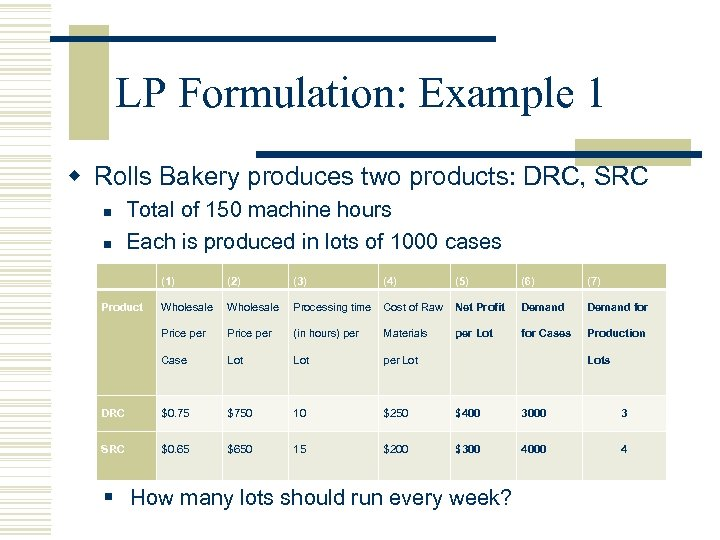LP Formulation: Example 1 w Rolls Bakery produces two products: DRC, SRC n n Total of 150 machine hours Each is produced in lots of 1000 cases (1) (2) (3) (4) (5) (6) (7) Product Net Profit Demand Product Wholesale Processing time Cost of Raw Net Wholesale Processing Cost of Demand for Price per per (in hours) per Price time Materials Raw per Lot Profit for Case per Lot Materials per Lot Lot Lot (in hours) per Lot for Cases Production Cases Lots DRC \$0. 75 \$750 10 10 \$250 ? \$400 ? 3000 ? 3 SRC \$0. 65 \$650 15 15 \$200 ? \$300 ? 4000 ? 4 § How many lots should run every week?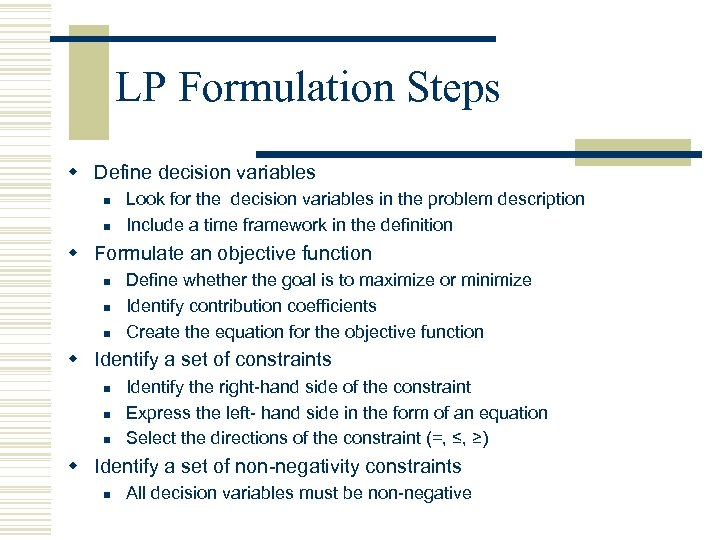LP Formulation Steps w Define decision variables n n Look for the decision variables in the problem description Include a time framework in the definition w Formulate an objective function n Define whether the goal is to maximize or minimize Identify contribution coefficients Create the equation for the objective function w Identify a set of constraints n n n Identify the right-hand side of the constraint Express the left- hand side in the form of an equation Select the directions of the constraint (=, ≤, ≥) w Identify a set of non-negativity constraints n All decision variables must be non-negative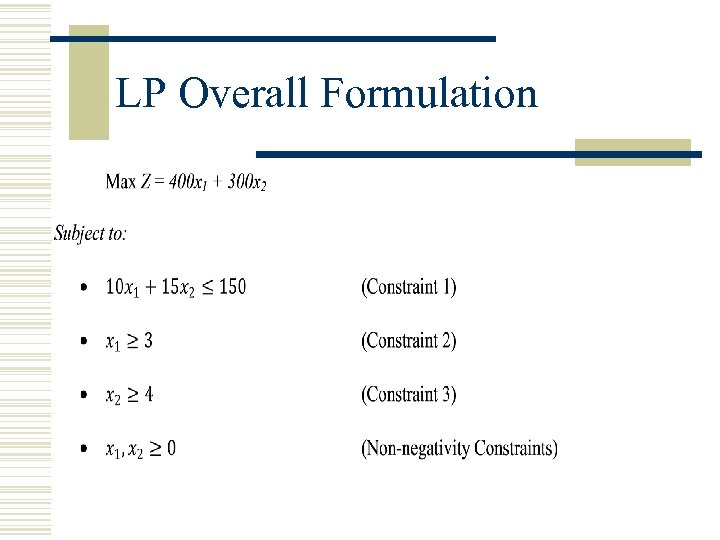LP Overall Formulation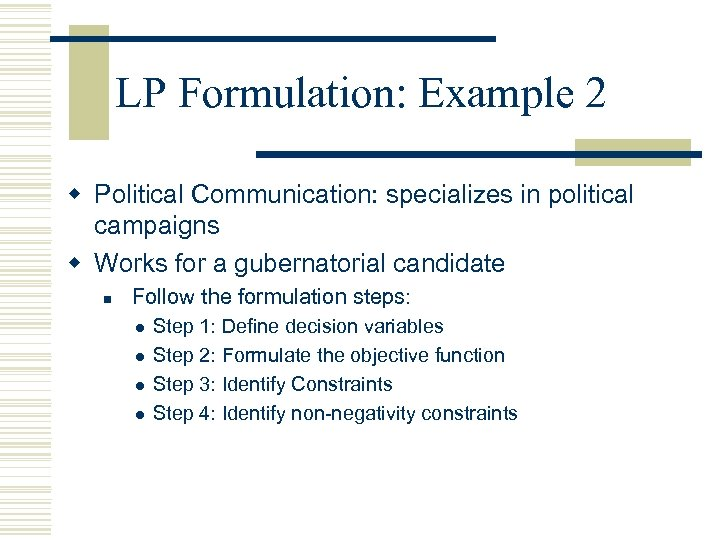LP Formulation: Example 2 w Political Communication: specializes in political campaigns w Works for a gubernatorial candidate n Follow the formulation steps: l l Step 1: Define decision variables Step 2: Formulate the objective function Step 3: Identify Constraints Step 4: Identify non-negativity constraints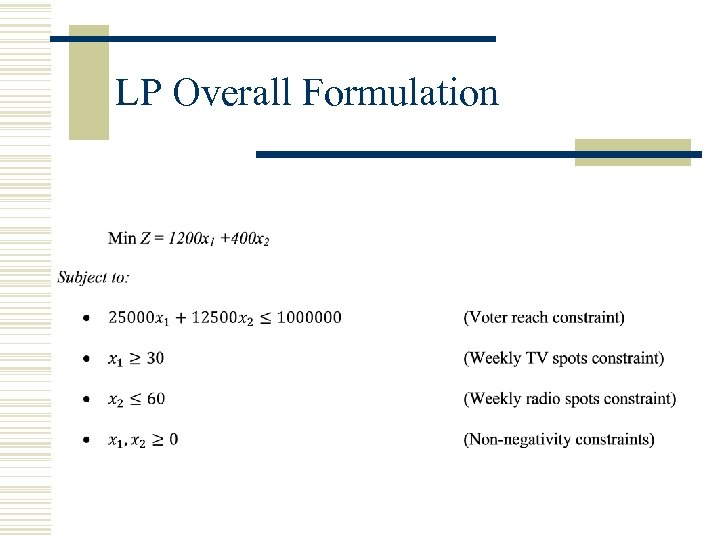LP Overall Formulation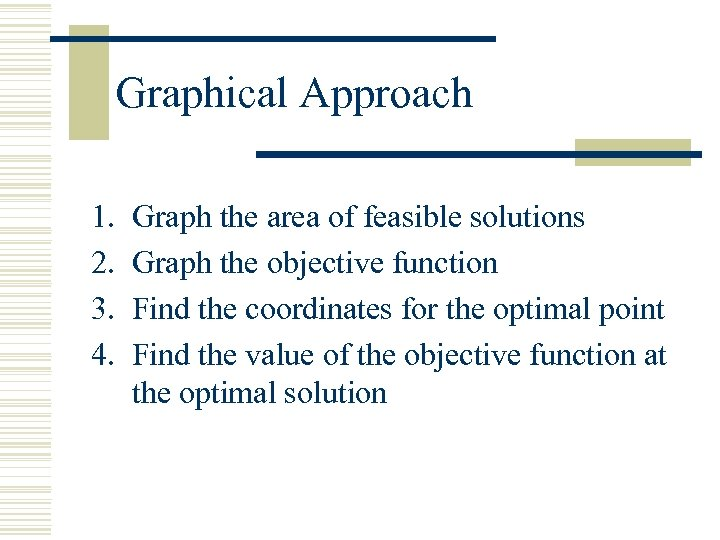Graphical Approach 1. 2. 3. 4. Graph the area of feasible solutions Graph the objective function Find the coordinates for the optimal point Find the value of the objective function at the optimal solution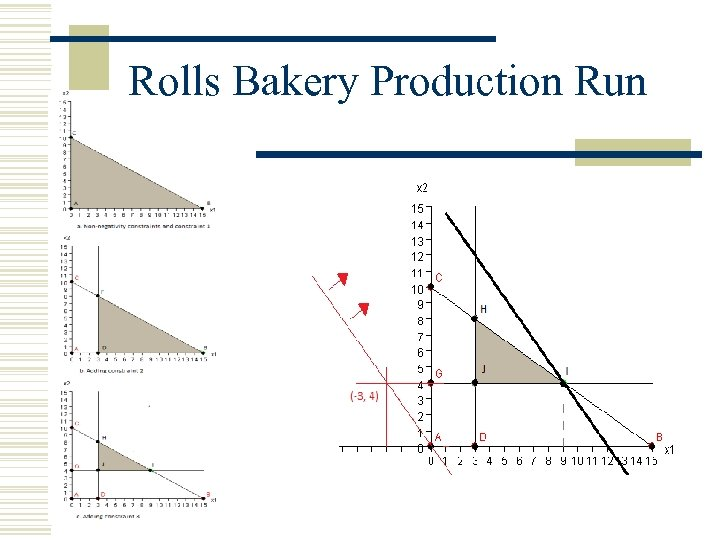Rolls Bakery Production Run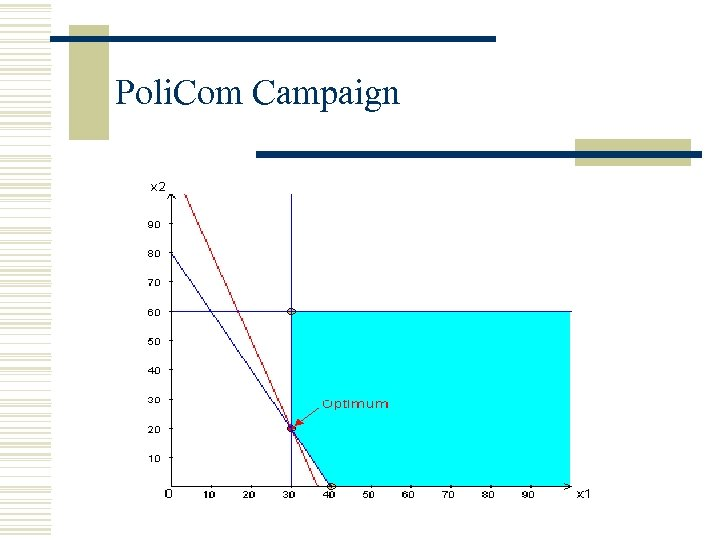Poli. Com Campaign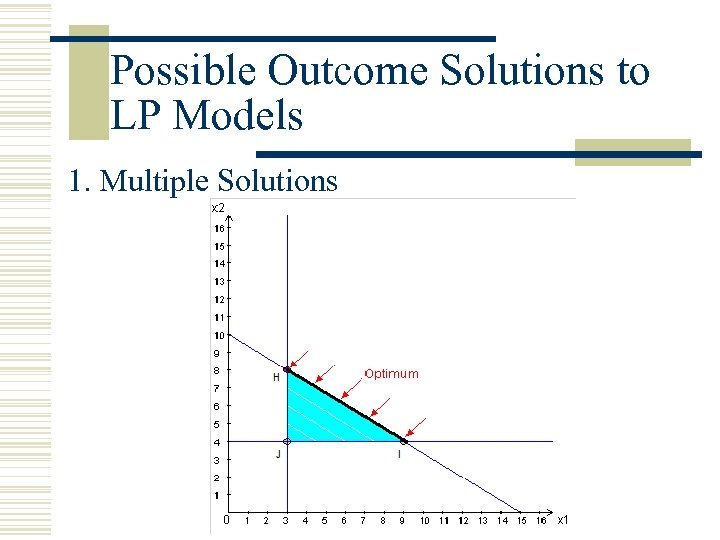Possible Outcome Solutions to LP Models 1. Multiple Solutions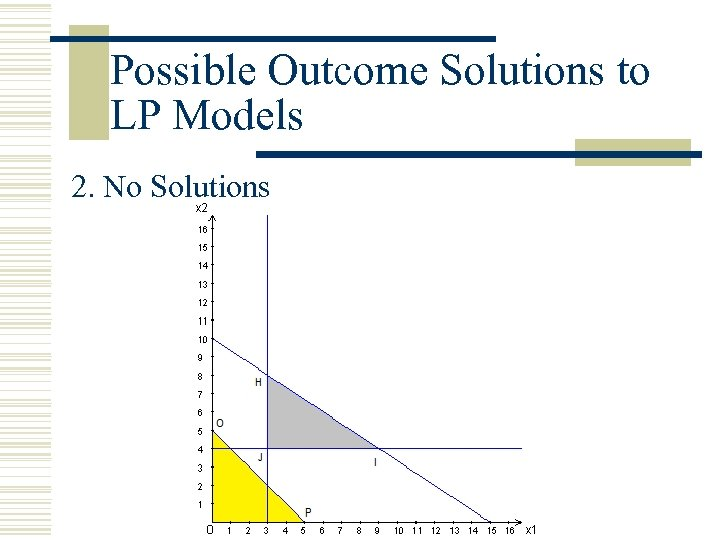Possible Outcome Solutions to LP Models 2. No Solutions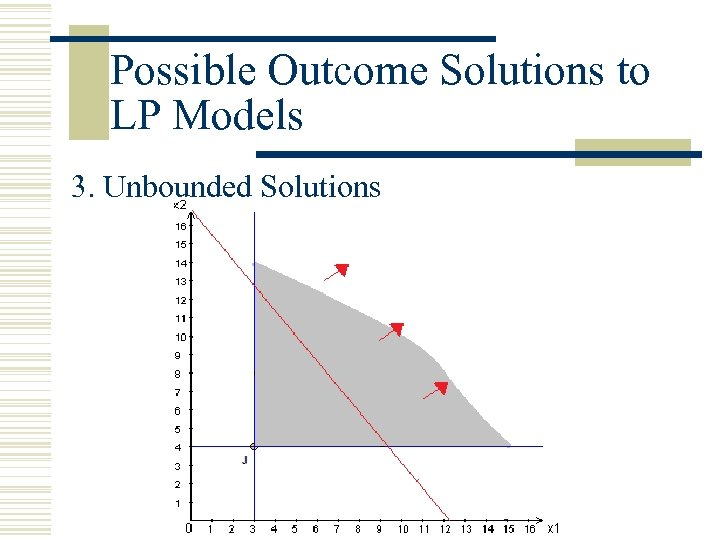Possible Outcome Solutions to LP Models 3. Unbounded Solutions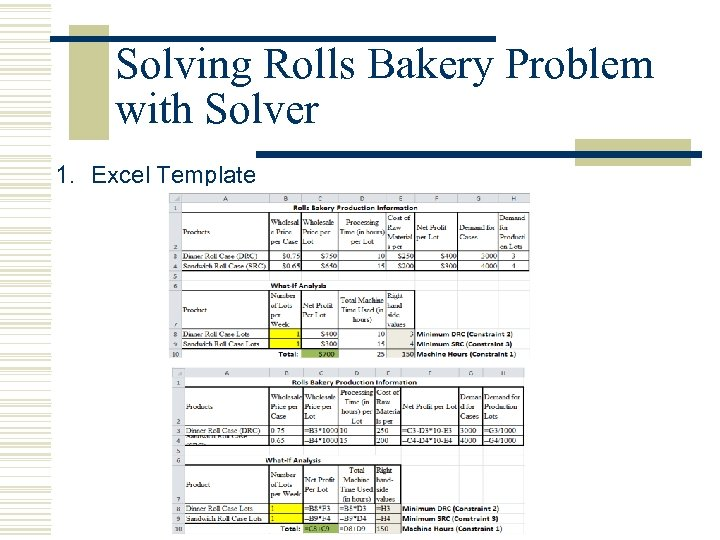Solving Rolls Bakery Problem with Solver 1. Excel Template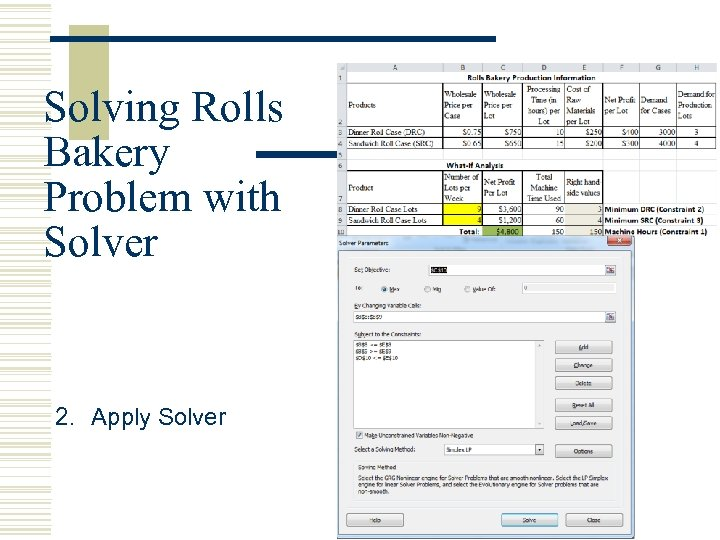Solving Rolls Bakery Problem with Solver 2. Apply Solver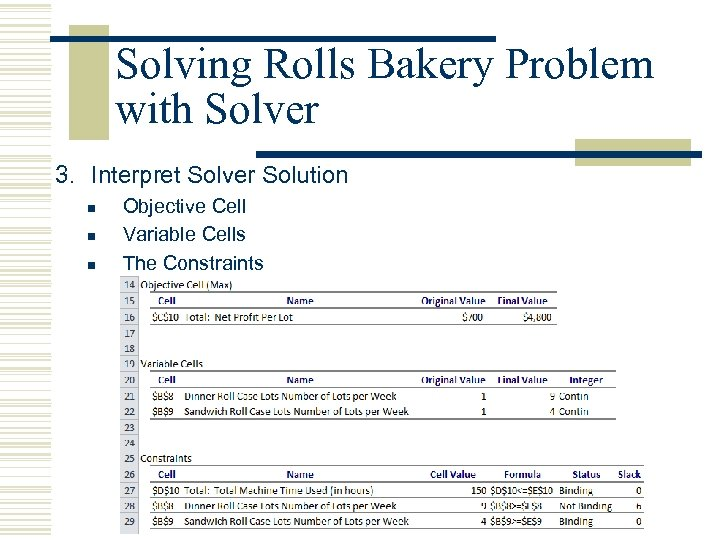Solving Rolls Bakery Problem with Solver 3. Interpret Solver Solution n Objective Cell Variable Cells The Constraints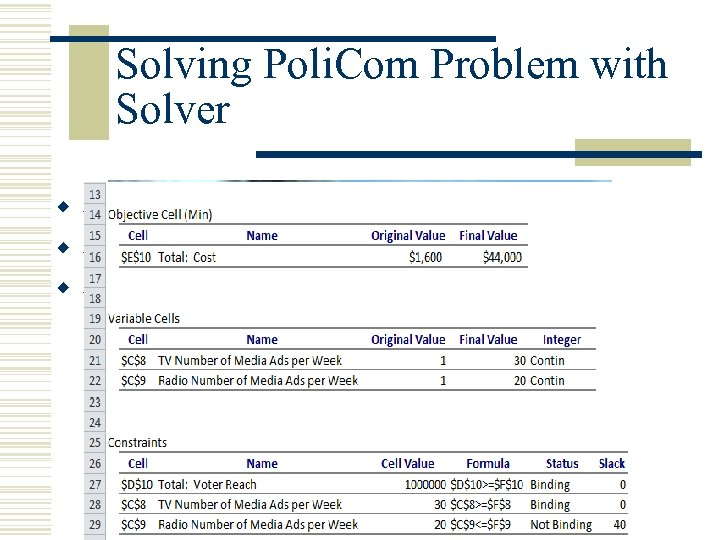Solving Poli. Com Problem with Solver w Stage 1: Create a Template w Stage 2: Apply Solver w Stage 3: Interpret Solver Solution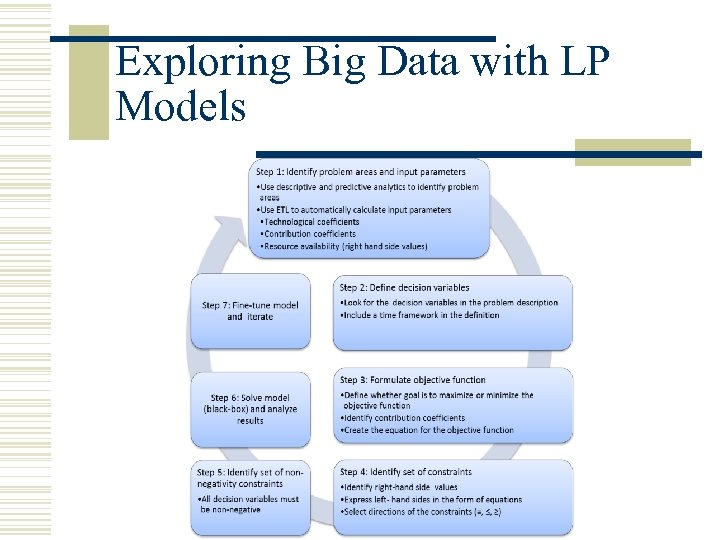Exploring Big Data with LP Models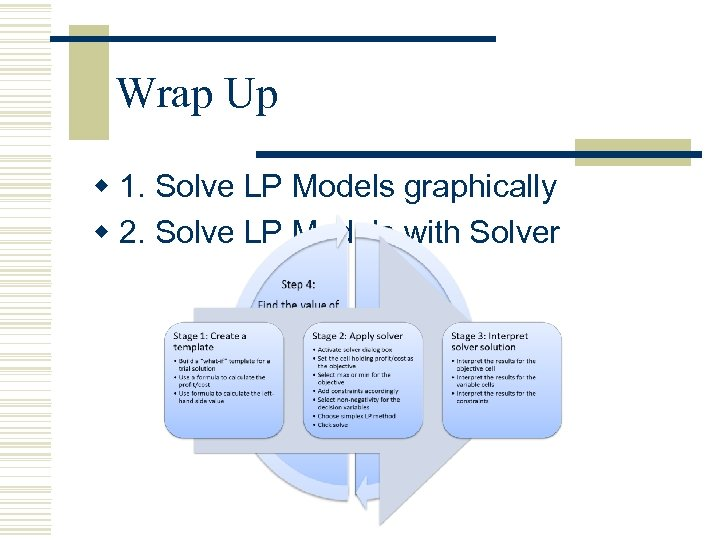Wrap Up w 1. Solve LP Models graphically w 2. Solve LP Models with Solver